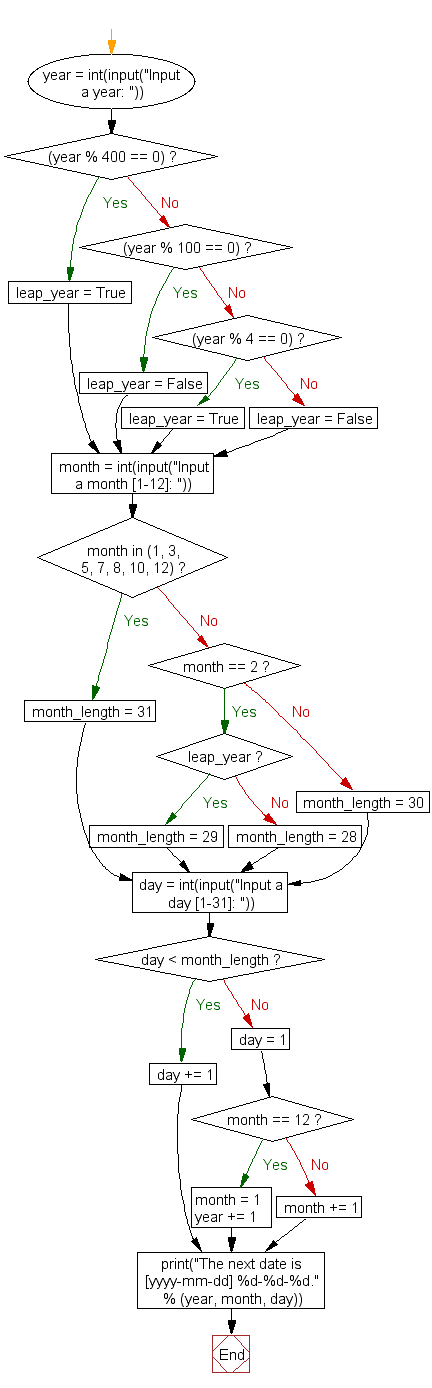﻿ Python Exercise: Get next day of a given date - w3resource# Python Exercise: Get next day of a given date

## Python Conditional: Exercise - 41 with Solution

Write a Python program to get next day of a given date.

Sample Solution:

Python Code:

``````year = int(input("Input a year: "))

if (year % 400 == 0):
leap_year = True
elif (year % 100 == 0):
leap_year = False
elif (year % 4 == 0):
leap_year = True
else:
leap_year = False

month = int(input("Input a month [1-12]: "))

if month in (1, 3, 5, 7, 8, 10, 12):
month_length = 31
elif month == 2:
if leap_year:
month_length = 29
else:
month_length = 28
else:
month_length = 30

day = int(input("Input a day [1-31]: "))

if day < month_length:
day += 1
else:
day = 1
if month == 12:
month = 1
year += 1
else:
month += 1
print("The next date is [yyyy-mm-dd] %d-%d-%d." % (year, month, day))
```
```

Sample Output:

```Input a year: 1974
Input a month [1-12]: 2
Input a day [1-31]: 15
The next date is [yyyy-mm-dd] 1974-2-16.
```

Flowchart :## Visualize Python code execution:

The following tool visualize what the computer is doing step-by-step as it executes the said program:

Python Code Editor:

Have another way to solve this solution? Contribute your code (and comments) through Disqus.

What is the difficulty level of this exercise?

Test your Python skills with w3resource's quiz

﻿

## Python: Tips of the Day

Function argument unpacking in Python.

Example:

```def tips_func(x, y, z):
print(x, y, z)

tuple_val = (2, 0, 2)
dict_val = {'x': 3, 'y': 2, 'z': 1}
tips_func(*tuple_val)
tips_func(**dict_val)
```

Output:

```2 0 2
3 2 1
```